# Standard Surge Waveforms

Wavelet transformation can be either continuous or discrete.  However, the Discrete Wavelet Transformation (DWT) is more widely used, chiefly due to its computational efficiency and data compression capability. The following is a brief description of wavelet transformation theory.

## IEEE/IEC

The standard waveshape for the surge, according to references  and , can be written in terms of two exponentials,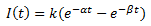(1)

Where k, α, β are constants and t is time in microseconds. The typical surge waveform is show below: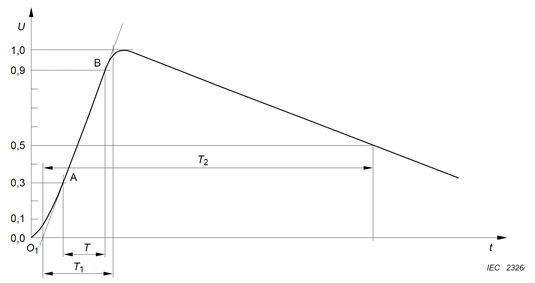Typical Surge Waveform

In power system studies, the surge waveform is usually defined in terms of wavefront time (Tf)  and wavetail time (Tt).  As seen in the figure above, wavetail time is the time when the current waveform reaches half of its maximum value (i.e. Tt = T2).  Similarly the wavefront time (or rise time) is defined as 1.67(T0.9 - T0.1) or 1.25(T0.9 - T0.3). The factor k in (1) is selected such that the maximum voltage is unity.

### Standard, Commonly Used Waveforms

The following values were generated using the MATLAB optimization algorithm (except for the 8/20 us waveform):

 Waveform Reference Wavefront and Wavetail Basis Parameters of the Waveform a b k 1.2/50 IEC 61000-4-5 IEC 60060-1 T1 = 1.67T T2 = 50 0.0145 2.8353 1.0328 8/20 IEC 61000-4-5 IEC 60060-1 T1 = 1.25T T2 = 50 0.1732 0.0866 4 1/50 IEC 69469-1 T1 = 1.25T T2 = 501 0.014576 2.5293 1.0363 250/2500 T1 = 1.25T T2 = 2500 0.00035239 0.0080519 1.2068 250/1500 T1 = 1.25T T2 = 1500 0.00072065 0.0065795 1.4741 10/700 IEC 60060-1 T1 = 1.67T T2 = 700 0.0010222 0.3063 1.0227 5/320 IEC 60060-1 T1 = 1.25T T2 = 320 0.0022548 0.51343 1.0288

### Custom Waveforms

Method 1: Using α and β values as in equation (1). The k factor is defined as: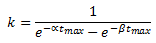(2)

Where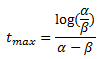(3)

Method 2: Construction of the waveform based on wavefront and wavetail times. This is an approximate method. It is assumed that α << β and k ≈ 1. Then, it can be shown that, the front-time (Tf, defined as 10% and 90% basis) is: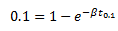,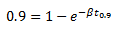(4)

And: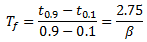(5)

If defined as 30% and 90% basis: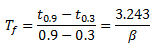(6)

Similarly the wavetail time Tt is: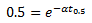,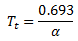(7)

Once α and β  are defined, the surge waveform is: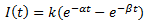(8)

EXAMPLE:

The following figure compares the approximate waveform using the above method and the accurate solution obtained using the MATLAB optimization algorithm for the 1.2/50 us surge waveform.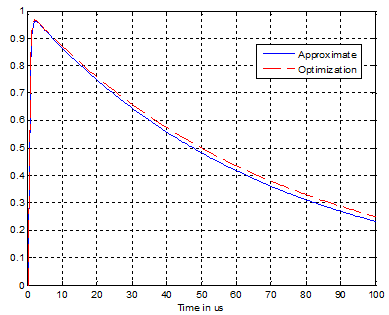## CIGRÉ

The CIGRÉ standard waveform is defined as follows.

For the current front: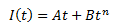(9)

For the current tail: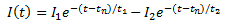(10)

The constants are found in reference .